# Electric Machines Laboratory

ELECTRIC MACHINES LABORATORY

The Electric Machines Laboratory at the Faculty of Engineering, University of Jaffna has its purpose to provide education, research, and service in all aspects of electrical power generation and energy conversion. The Laboratory is equipped with the Three major types of electric machines: Induction machines, Synchronous machines, DC Machines, and Transformers.

As well as tachometers, variable, and constant AC and DC supply and with meters to measure voltages, currents, power and speed. The lab is primarily used to teach and demonstrate the many configurations of machines, transformers.

Synchronous Generator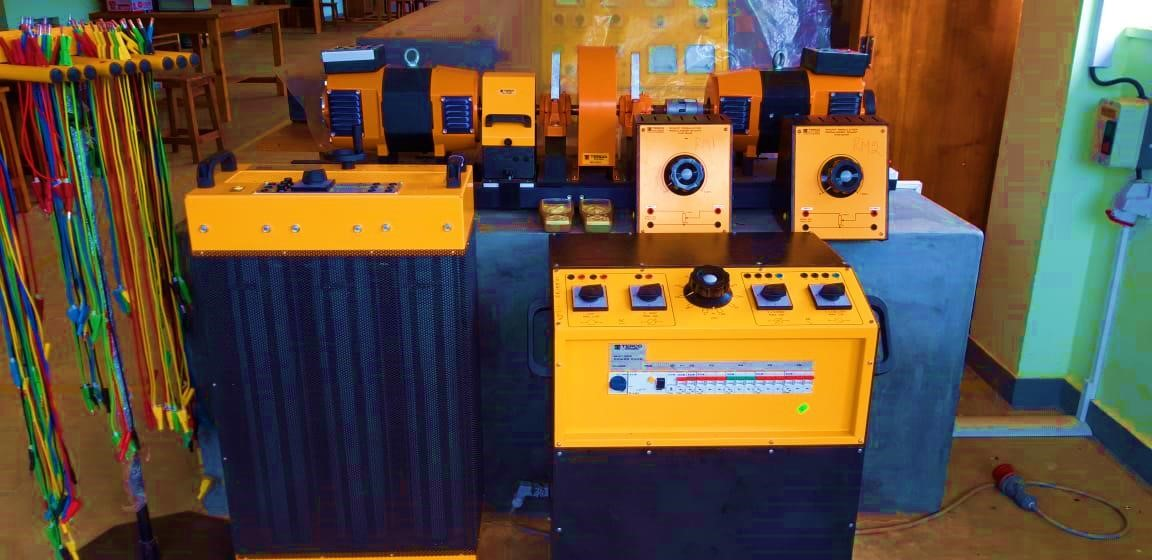By performing the Synchronous Generator lab experiment students can learn how to wire the measurement stand, perform the experiment by applying variable supply voltage and variable load, and study the performance of Synchronous machine in steady-state conditions.
The main purpose of this lab is to understand the no-load characteristics, the short-circuit characteristics, and the load characteristics of a synchronous generator.

Asynchronous Wind Mill System

By demonstrating this setup students can able to get fundamental understanding of the different essential branches of the AWMS such as:

• Working characteristics depending on resistive loads
• The influence of compensating inductances
• Total efficiency depending on involved parameters
• Principles of floating speed and frequency
• Principles of DC energy transfer using a 4Q-converter operating in current limit mode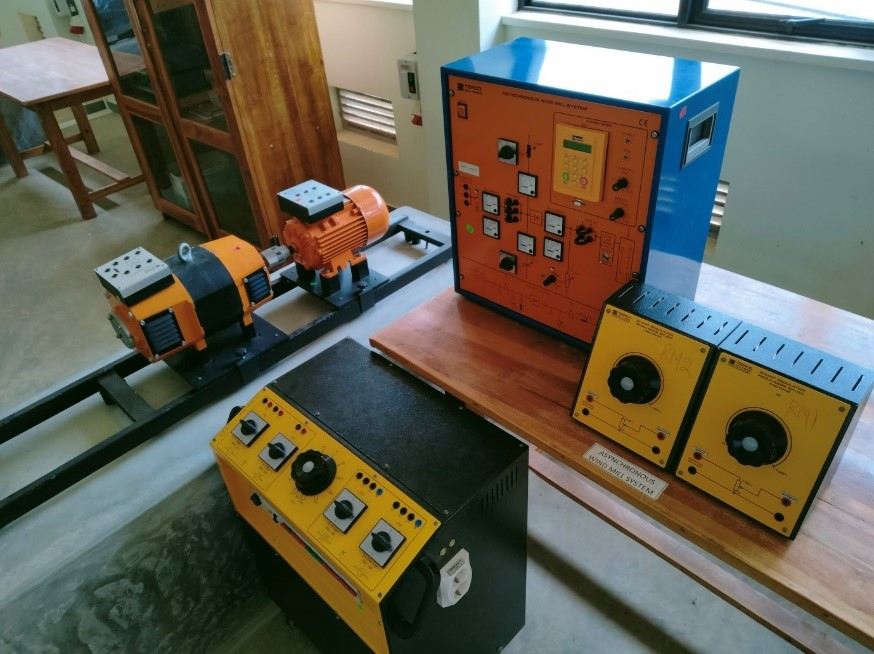Salient Pole Motor

This laboratory setup offers the opportunity to learn and understand the characteristic concept of a unique salient pole rotor-type motor. It provides an understanding of physical magnitudes, and the opportunity to examine elements of system behavior which are not explained by idealized theory.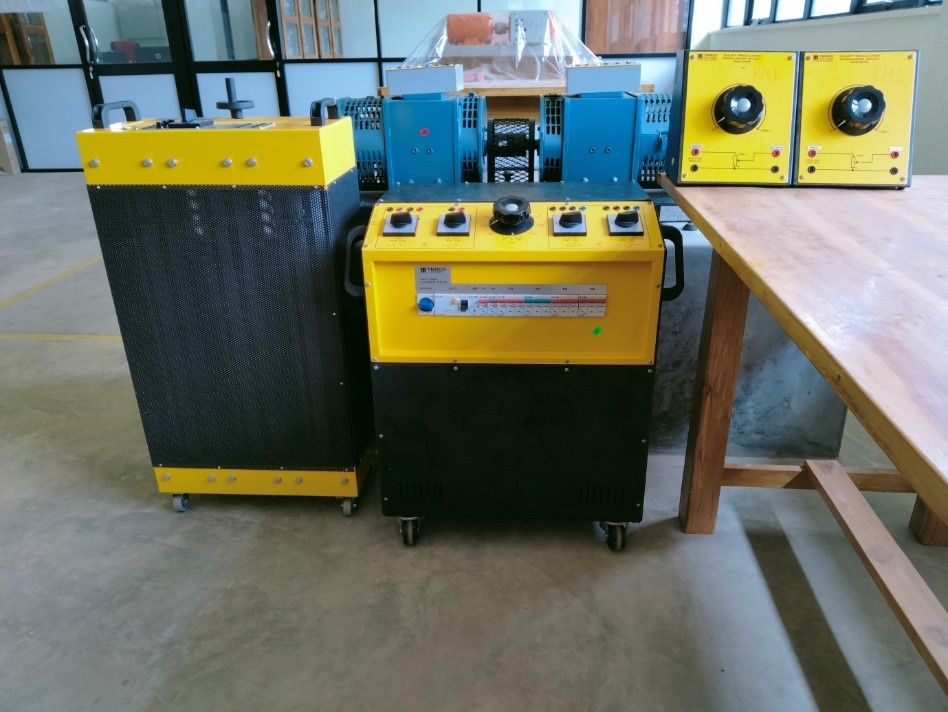Three Phase Transformer

This transformer experiment provides chance to students to get hands on experience with real three phase transformer through carried out testing. With this setup module we can perform the following studies.

• To conduct standard open and short circuit tests to ﬁnd the parameters of the equivalent circuit of a transformer.
• Evaluate the regulation and efficiency of the transformer at a given load.
• Check the excitation characteristics of the transformer.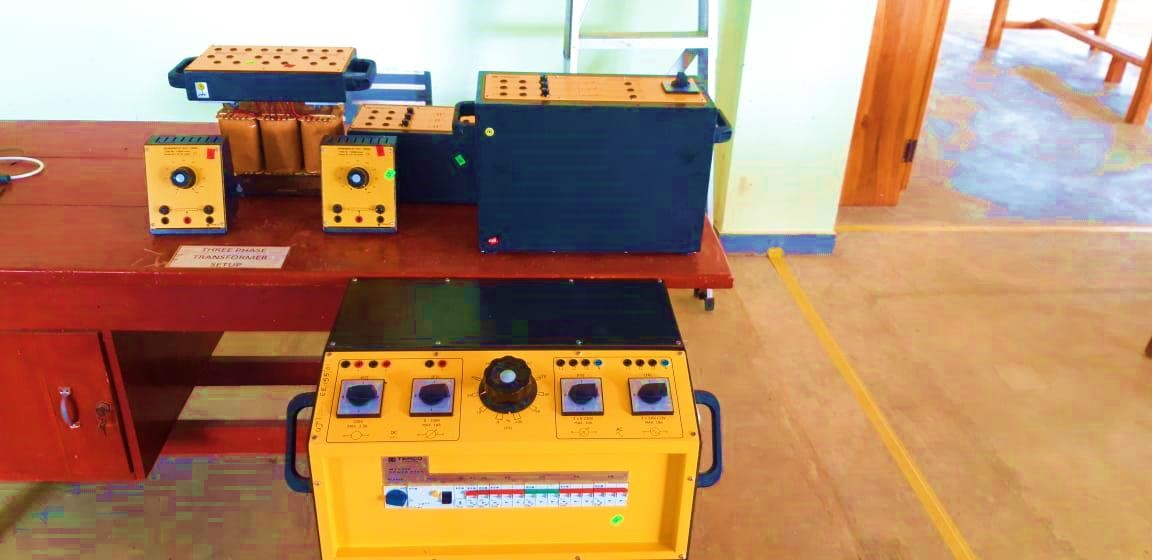Power Factor Control Unit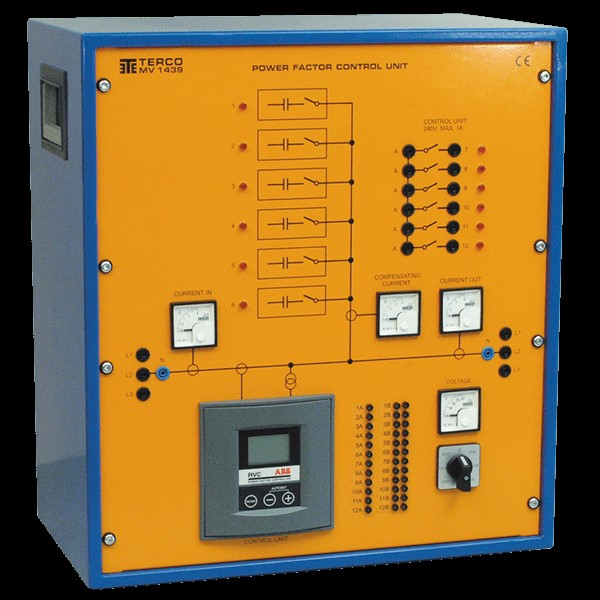PFC can be used to minimise the currents caused by reactive losses of power and thereby optimising the transfer of energy between generation and loading. This is becoming increasingly important today when “Saving energy” is vital in a world with focus on pollution and shortage of energy.

Field of application

Inductive or mixed inductive and resistive networks in need of compensation, for example when starting and running induction motors.

Principles of operation

Depending on the power factor of the loading network a microprocessor will connect groups of capacitors.

By measuring phase voltages and current the microprocessor will calculate how many capacitive groups that must be connected and in which combinations.

Solar PV Experiment Setup

A photovoltaic (PV) system is composed of one or more solar panels combined with a variable load set.

Student would be able to understand the following Basic concept of PV systems by demonstrating this laboratory session.

• Analyzing the current-voltage characteristic of solar PV system.
• Analyzing irradiation and temperature effects on PV solar system.
• Finding the maximum power point of PV system.

Induction Machine Setup

Studies conducted using this setup: current – torque characteristics, torque- Power characteristics and torque – speed characteristics of a squirrel cage induction Machine.